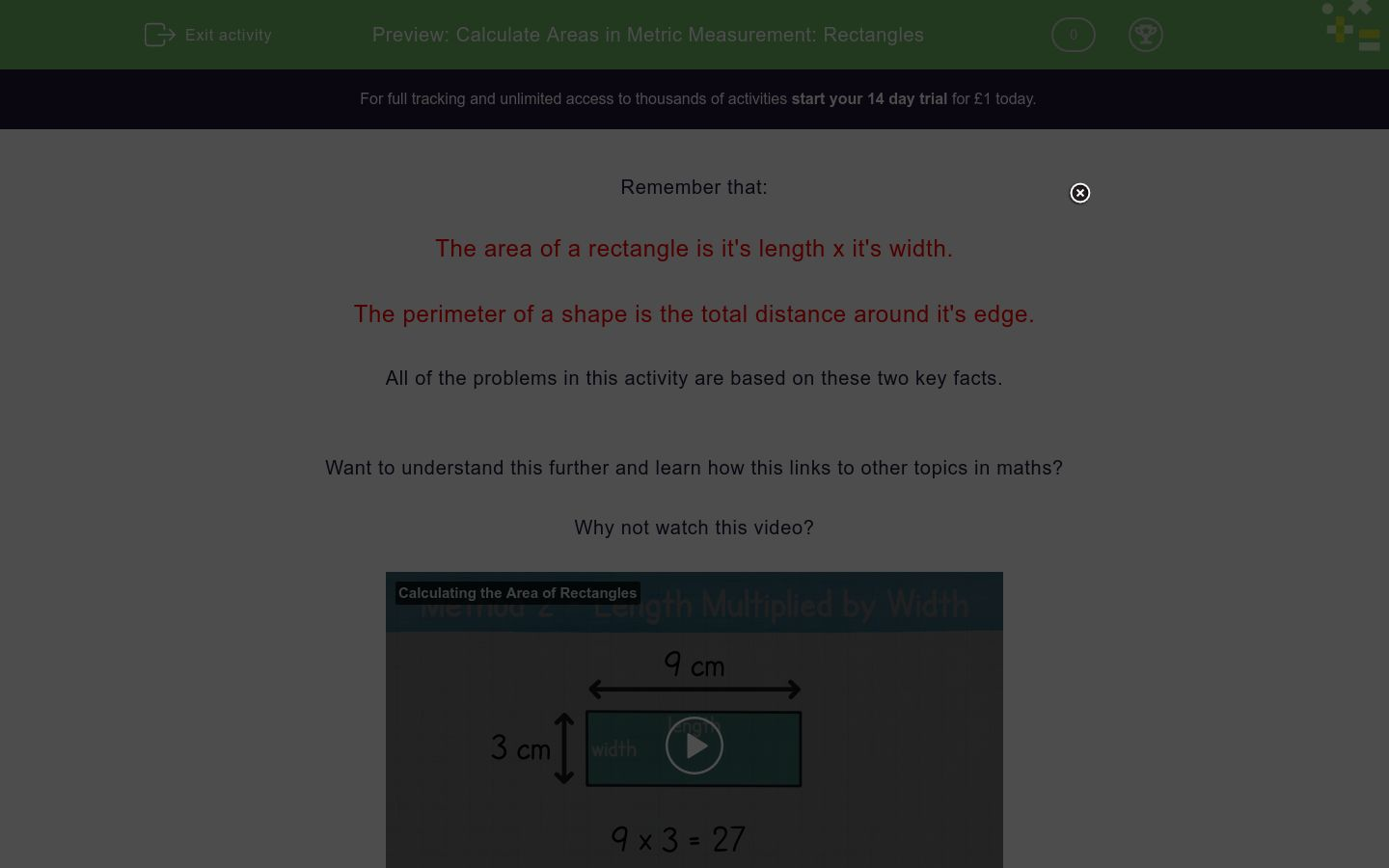# Calculate Areas in Metric Measurement: Rectangles

In this worksheet, students calculate the area and perimeter of rectangles using metric measurements.Key stage:  KS 2

Curriculum topic:   Measurement

Curriculum subtopic:   Calculate/Estimate Areas

Difficulty level:### QUESTION 1 of 10

Remember that:

The area of a rectangle is it's length x it's width.

The perimeter of a shape is the total distance around it's edge.

All of the problems in this activity are based on these two key facts.

Want to understand this further and learn how this links to other topics in maths?

Why not watch this video?

A rectangular field measures 50m by 40m.

What is its area in square metres?

(Just write the number)

A rectangular field measures 50m by 40m.

What is its perimeter in metres?

(Just write the number)

A rectangular field is 40m wide.  It is three times as long as it is wide.

What is its perimeter in metres?

(Just write the number)

A rectangular field is 40m wide.  It is three times as long as it is wide.

What is its area in square metres?

(Just write the number)

A rectangular field has a perimeter of 100m.  It is 20m wide.

What is its length in metres?

(Just write the number)

A rectangular field has a perimeter of 100m.  It is 20m wide.

What is its area in square metres?

(Just write the number)

A rectangular field has a perimeter of 140m.  It is 20m wide.

What is its area in square metres?

(Just write the number)

A rectangular field has a perimeter of 150m.  It is 20m wide.

What is its area in square metres?

(Just write the number)

A rectangular field has an area of 1600m2.  It is 20m wide.

What is its perimeter in metres?

(Just write the number)

A rectangular field has an area of 1600m2.  It is 50m wide

What is its perimeter in metres?

(Just write the number)

• Question 1

A rectangular field measures 50m by 40m.

What is its area in square metres?

(Just write the number)

2000
EDDIE SAYS
50 x 40 = 2000
• Question 2

A rectangular field measures 50m by 40m.

What is its perimeter in metres?

(Just write the number)

180
EDDIE SAYS
50 + 50 + 40 + 40 = 180
• Question 3

A rectangular field is 40m wide.  It is three times as long as it is wide.

What is its perimeter in metres?

(Just write the number)

320
EDDIE SAYS
40 x 3 = 120 (three times as long as it is wide) 40 + 40 + 120 + 120 = 320
• Question 4

A rectangular field is 40m wide.  It is three times as long as it is wide.

What is its area in square metres?

(Just write the number)

4800
EDDIE SAYS
40 x 120 = 4800
• Question 5

A rectangular field has a perimeter of 100m.  It is 20m wide.

What is its length in metres?

(Just write the number)

30
EDDIE SAYS
The width is 20 + 20 = 40. Subtract the perimeter and the width to get the length 100 - 40 = 60. The length of one side is 60 divided by 2 = 30.
• Question 6

A rectangular field has a perimeter of 100m.  It is 20m wide.

What is its area in square metres?

(Just write the number)

600
EDDIE SAYS
Area = length x width Area = 30 x 20 = 600 (We found the length in the last question. The width is 20 + 20 = 40. Subtract the perimeter and the width to get the length 100 - 40 = 60. The length of one side is 60 divided by 2 = 30.)
• Question 7

A rectangular field has a perimeter of 140m.  It is 20m wide.

What is its area in square metres?

(Just write the number)

1000
EDDIE SAYS
The width is 20 + 20 = 40. Subtract the perimeter and the width to get the length 140 - 40 = 100. The length of one side is 100 divided by 2 = 50. Area = length x width Area = 50 x 20 = 1000
• Question 8

A rectangular field has a perimeter of 150m.  It is 20m wide.

What is its area in square metres?

(Just write the number)

1100
EDDIE SAYS
The width is 20 + 20 = 40. Subtract the perimeter and the width to get the length 150 - 40 = 110. The length of one side is 110 divided by 2 = 55. A = l x w A = 55 x 20 = 1100
• Question 9

A rectangular field has an area of 1600m2.  It is 20m wide.

What is its perimeter in metres?

(Just write the number)

200
EDDIE SAYS
A = length x width 1600 = l x 20 1600/20 = 80 is the length Width = 20 x 2 = 40 Length = 80 x 2 = 160 Perimeter = 160 + 40 = 200
• Question 10

A rectangular field has an area of 1600m2.  It is 50m wide

What is its perimeter in metres?

(Just write the number)

164
EDDIE SAYS
A = l x w 1600 = l x 50 1600/50 = 32 is the length Width = 50 x 2 = 100 Length = 32 x 2 = 64 Perimeter = 100 + 64 = 164
---- OR ----

Sign up for a £1 trial so you can track and measure your child's progress on this activity.

### What is EdPlace?

We're your National Curriculum aligned online education content provider helping each child succeed in English, maths and science from year 1 to GCSE. With an EdPlace account you’ll be able to track and measure progress, helping each child achieve their best. We build confidence and attainment by personalising each child’s learning at a level that suits them.

Get started